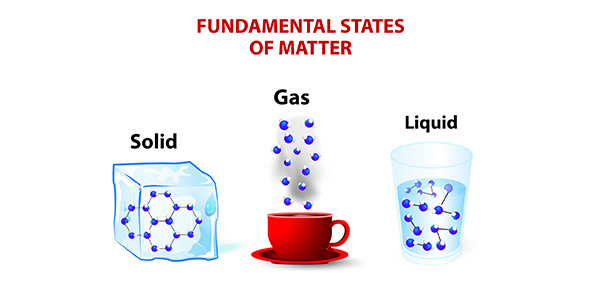# Matter

20 QuestionsSettingsRelated Topics
• 1.
Matter is something that has
• A.

Mass & Neutrons

• B.

Mass & Weight

• C.

Volume & Solubility

• D.

Mass & Volume

• 2.
Which of the following is NOT a state of matter?
• A.

Solid

• B.

Liquid

• C.

Gas

• D.

Plasma

• E.

All are states of matter

• 3.
If an ice cube suddenly turned into water vapor this process would be known as
• A.

Sublimation

• B.

Condensation

• C.

Freezing

• D.

Vaporization

• 4.
A man takes a piece of paper and burns it so that it turns into ash and smoke.  This is known as a
• A.

Physical change

• B.

Chemical change

• 5.
When the chemical composition of matter changes so that it turns into a new type of matter, it is known as a
• A.

Physical change

• B.

Chemical change

• 6.
If wood is burned and changed into ash and smoke, then what would be the mass of the smoke if the wood was 30 grams and the ash was 10 grams?
• A.

40 grams

• B.

20 grams

• C.

3 grams

• D.

300 grams

• 7.
What sub atomic particle has a positive charge?
• A.

Protons

• B.

Neutrons

• C.

Electrons

• D.

Nucleus

• 8.
Which element has an atomic number of 26?
• A.

Aluminum

• B.

Iron

• C.

Scandium

• D.

Cobalt

• 9.
How many electrons deos Zinc have?
• A.

30

• B.

35

• C.

36

• D.

31

• 10.
Which element has 20 neutrons?
• A.

Sodium

• B.

Copper

• C.

Fluorine

• D.

Potassium

• 11.
Helium js considered to be a
• A.

Metal

• B.

Nonmetal

• C.

Metalloid

• 12.
Identify the element that conducts electricity the best.
• A.

Magnesium

• B.

Oxygen

• C.

Boron

• D.

Phosphorus

• 13.
Name the element that is located in Group 4, Period 5.
• A.

• B.

Titanium

• C.

Zirconium

• D.

Tin

• 14.
When an element has the same number of protons but a different amount of neutrons, then it is called a(n)
• A.

Substance

• B.

Isotope

• C.

Compound

• D.

Mixture

• 15.
When salt is combined with water it is known as a
• A.

Homogenous mixture

• B.

Heterogeneous mixture

• C.

Compound

• D.

Element

• 16.
When sugar is added to water, the sugar is known as the
• A.

Solute

• B.

Solvent

• C.

Solution

• 17.
As temperature increases, what usually happens to the solubility?
• A.

It increases

• B.

It decreases

• C.

It does not change

• 18.
Name a reactant in the process of photosynthesis.
• A.

Sugar

• B.

Oxygen

• C.

Carbon dioxide

• D.

ATP

• 19.
What does Exothermic mean?
• A.

Radiant energy is being absorbed in the process

• B.

Radiant energy is being given off from the process

• C.

Thermal energy is being absorbed in the process

• D.

Thermal energy is being given off from the process

• 20.
Which is true about a catalyst?
• A.

It slows down the rate of a chemical reaction

• B.

It speeds up the rate of a chemical reaction

• C.

It causes a chemical reaction to stop

• D.

It makes a chemical reaction reversible.